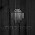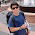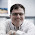### Create QR Codes in Excel (or any Spreadsheet)

For a little project I'm working on, I needed to understand how to generate QR codes. Being the Excel junkie that I am, I thought it would be easy to create a proof-of-concept entirely in Excel. As a challenge, I set a goal of doing this without using any Visual Basic or other scripting.

If you just want to generate the QR codes and not worry about the details of how it's done, just download the spreadsheet by clicking here and you can just use it standalone. Everything you need is in the tab "QR Code." Just enter text (up to 15 characters long) in the yellow box (Cell Y36) and the codes will be generated.

If you want to know how this all works, below is some explanation. To follow along, go to the "Generate QR" tab.

There are many sizes of QR codes, the smallest being a 21x21 pixel matrix known as Version 1 and the version that I have created in this spreadsheet.

To follow along, I will assume that you have not modified the original spreadsheet and "This is a test" is the string that we will encode into QR.

Step 1: There are several "Modes" you can use, but for the purposes of this spreadsheet we will use the "AlphaNumeric" mode. You can see that this is encoded with a 0010. For reasons I will explain in step 2, the spreadsheet will need to be modified if you want to use a different mode.

Step 2: We need to encode the overall length of the message. In a Version 1 AlphaNumeric QR code (21x21 pixels) there is only enough room for a maximum of 15 characters. Cell D4 has the text we want to encode and cell C7 is simply the length of the string displayed in 9-bit binary. "This is a test" contains 14 characters (including spaces). The number 14 in binary is 1110 and because we need to represent this as a 9-bit character, we put 0s to the left so that there are 9 characters. This is very easy to do in Excel with the DEC2BIN function. As I mentioned in Step 1, if you want to use another mode (Numeric, Binary or Japanese) you will need to modify Step 2 since they use a different number of bits. Numeric uses 10 bits, while Binary and Japanese use 8.

Step 3: We now need to encode the message itself. To do this, we break the message into sets of 2 characters (i.e. TH, IS,I, S, A, TE, ST). We also assign each letter a numeric value (as defined in the table I have placed in the Character Map sheet). For each pair of characters, we take the numeric value of the first character and multiply it by 45. Then we add that number to the numeric value of the second character. We then convert the result into an 11-bit binary string. If you are encoding an odd number of characters, then take the ASCII value of the final character and convert it into a 6-bit binary string. For the other modes (numeric, binary, and Japanese,) we would use a different method to encode the data. In Excel, I've done this by breaking out each character of the message in column G using the various text functions. Column H helps to tell us if it's an even or odd character (so we know if we need to multiply by 45) and column I has the numeric code that corresponds to each character using a lookup in the character map table. Column M helps us find which is the last row while Column N adds up the pair (or if there isn't a pair due to an odd number of characters, then just takes the last value). We need to convert the decimal number in column N to either an 11-bit or 6-bit binary number which I would ordinarily do with the DEC2BIN function. Unfortunately, Excel cannot do DEC2BIN on decimal numbers larger than 511. So, in Column O you can see the workaround that I had to use by breaking it down into small chunks and then putting it back together.

Step 4: Here we simply combine the strings from steps 1, 2 and 3. There is an IF/THEN function in there to handle the cases where the message we want to encode (e.g. "This is a test") has an even or odd number of characters.

Step 5: We now need to make sure that we have the correct length of string. QR codes have in-built error correction and there are different levels you can use. We will use Level Q. For this we need to make sure that we create a string with a total length of 104 bits. In Step 5 we add up to four 0s to lengthen the string. In our case, the length of the string in Step 4 was 90 characters, so we add four 0s to the end. If the length of the string in Step 4 was 102 characters, then we would only add two 0s to the end. This is done in Excel with a series of IF/THEN functions.

Step 6: If by the end of step 5 we still don't have the correct length (104 bits) then we need to continue padding it out. The way to do this is to break the string from Step 5 into 8-bit words. We will need 13 of these 8-bit words (8x13=104) by the time we're done. Column AB has the string from Step 5 broken into 8-bit words. The first thing to do is to add 0s to the end of the last word to make sure all words from Step 5 have 8 bits. You can see this in column AC. Then we keep adding new words (11101100 and 00010001) repeating in that order until we have 13. In column AD I identify which words need to be filled and then alternatively put Wordfill1 and Wordfill2. Column AE finishes it off by actually inserting the fill words.

Step 7: We need to convert the words into a "Message Polynomial" which is done by converting the binary words from Step 6 back into decimal. You can see this in column AG. Column AH has the exponent part which is shown here just for completeness, but is not used. In column AJ we convert the binary coefficient into what's known as Alpha notation

Step 8: Here we create a generator which is fixed for each version/type of QR code. The table in columns AM and AN are fixed values for the type of code we are creating (Version 1, AlphaNumeric mode with error correction level Q). For more info on how to create a generator for a different type of code, click here to search on Google.

Step 9: Here we perform the polynomial division using the message from Step 7 and the Generator from Step 8. Of interest here for the Excel implementation is that I had to create a method to repeatedly perform an XOR step. As I wrote at the beginning, I wanted to do all of this without writing any VBA code and there is no XOR function in Excel. You can see how I've implemented this in the XOR sheet.
NOTE: Step 9 has a known issue in it. The result of the XOR will always correctly give 0 as the first character. In some cases, the second (and subsequent) characters can also be a 0. If this is the case, then the MULT step must take the first non-zero digit. I have not implemented this and therefore the QR generator will fail under these circumstances. These are rare and hard to find occurrences, but if you want to try it out, trying to encode this string will fail: "2747585511"

Step 10: Phew, we've made it through all the tough math and it's pretty smooth sailing from here. The last column from Step 9 are the error correction words.

Step 11: We combine the first 13 words that we generated in step 6 and add the next 13 words from step 10. This is what we will be encoding into the QR code.

Step 12: Combining all the words together, we have our full binary string ready to populate into the QR matrix.

You can now switch to the QR Code sheet to see how we finish it off. I have copied the finished string from Step 12 to cell AW21 in the QR Code sheet for simplicity.

The QR code is drawn by turning pixels on or off. I have implemented this by using 1s and 0s in a matrix'ed set of cells (columns Y to AS). Using conditional formatting, I have set a 1 to be a black-filled cell and a 0 to be a white-filled cell. The trick is now to just create the matrix.

Step 13: There are some cells in the 21x21 matrix that always must be set in a particular way. These are the "position detection" cells and I have marked them off with a 1 and they are marked in green. There are also cells called "timing cells" which are always in a particular pattern. I have marked them in blue. We also need to load the data we created in Step 12 into the matrix. You can see in the matrix that stretches from cell B2 to V22, I have marked off where each bit goes. Starting at the lower left we put the first bit (that we created in Step 12), and move around the pattern as indicated. You can see how they all fit together in the matrix from B26 to V46.

Step 14: We're not quite done yet. Because the code we create could be difficult to read (e.g. if there are too many black pixels clustered together, etc.) we need to create 8 different versions of the code and select the best one based on a formula. To do this, we create a "mask" and filter the raw data (B26..V46) through it. Each mask is based on a formula which I will show you below. To tell the reader which mask is being used, we fill what I've labeled as the orange cells with a code to tell the reader which mask we're using. The table of codes can be seen in AV3..AW10. These are also repeated for the sake of ease down column AW at each code. You can see that in each block, the orange cells contain the bits we need to identify each mask.

Step 15: The matrices starting with B50..V70 (B74..V94, etc.) have been filled in with the mask formula. The mask is simply calculated as a function of the row/column of each cell.

1. If (row + column) mod 2 is 0
2. If (row) mod 2 is 0
3. If (column) mod 3 is 0
4. If (row + column) mod 3 is 0
5. If (floor (row / 2) + floor (column / 3) ) mod 2 is 0
6. If ((row * column) mod 2) + ((row * column) mod 3) is 0
7. If (((row * column) mod 2) + ((row * column) mod 3)) mod 2 is 0
8. If (((row + column) mod 2) + ((row * column) mod 3)) mod 2 is 0

If you look at the forumlas inside the mask matrices, you'll see how I've implemented them as Excel functions. Boolean functions in Excel normally return True/False, but if you multiply them by 1 you get 1/0 which is what we need.

Step 16: We now combine the raw data (Step 13) with the mask such that whenever the mask has a 1 we change the raw data to the opposite of what it is (from 1 to 0 or 0 to 1). Whenever the mask has a 0, we leave the raw data alone. The math to do this is quite easy and is done in the formulae hidden under the actual QR codes we've generated (Cells Y50..AS70, etc.). Using conditional formatting, we just set Excel to make the cells all white or all black based on the number underneath. As you can see, we create 8 QR codes (one for each mask). Officially, the next step is to pick the "best" one, but in practice, my iPhone QR reading app can read all of them... so find one that you like, test it and use it! If you really want to be compliant with the standard, you can Google how to pick the "best" pattern by clicking here.

1.Great to create and know the algorithm of QR code...
very information!
Thanx
Bala

2.3.This is impressive. I am actually looking for a way to use an Excel spreadsheet as my database from which to print QR codes. I'm looking for someone to do this for me. Is this something you would be interested in taking on? Or, do you have any suggestions for me? Basically, I want to put certain info into a database or a spreadsheet. This is for inventory of products and listing on a QR code which inventory is in each box that is packed. So, I'd like to list various pieces of information on the spreadsheet and have it set up in a program so that I can type in a Box ID, a 4 digit SKU of the inventory that I'm putting in the box, and the quantity of inventory I'm putting in the box. When complete I want it to print a QR code that includes another piece of information about the item and the quantity along with the PO number. Can you do this? Contact me at Mark@newgrowthpress.com if this is something you'd be interested in or if you know of someone else that could do it. Thanks!

4.That is really cool!!!!!!!!!!!!!!!!!!!!!!!!!!!!!!!!!!!

5.Realy nice work...congrats

6.Thank you !!
But I have one question. If I used this code "GH90-20865C", than the QR code gives me "GH90-25ZDT0", do you know why ? Coul you help me please ?

7.simply, WOW! good work!

8.Cool! great work!

9.Hello,
cool Tool. But at "E 10074" I get "E 10510" with the scanner. Where is the Problem?

10.hello. tanks for the information. but if i want to canges version 128. how to step this?

11.if I want to change the version. from version lv 1 to version lv 4 what should I change? tank you master.

12.I am using this and it's great.
http://technitya.com/barcode-generator-excel/

13.very impressive work!

14.This is fantastic, thank you very much! I'm having an issue with any code that has double 0's (600149). Anything that follows the 0's is not correct. Any ideas?

15.It is quite impressive work to understand QR coding process. Thank you!
It also illustrates how hard to debug this project in such level. I feed the QR Code'!Y36 cell with
'*+ blog +*
there is no warning/error, however, it totally codes a different string!

16.Wow... as someone who likes to do Excel stuff without using VBA, this totally trumps my puny QR effort! https://www.gamasutra.com/blogs/CBel/20180213/308549/3D_engine_entirely_made_of_MS_Excel_formulae__Enjoy_this_Doomxls_file_.php#comments